# Precalculus : Convert Polar Equations To Rectangular Form and vice versa

## Example Questions

1 2 3 4 6 Next →

### Example Question #121 : Polar Coordinates And Complex Numbers

Write the equation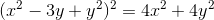in polar form

Possible Answers: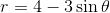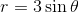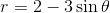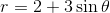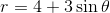Correct answer:Explanation:

First re-arrange the original equation so that the 4 is factored out on the right side, and putand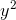next to each other: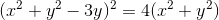Make the substitutions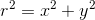and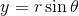: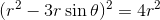take the square root of both sides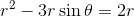divide both sides by r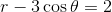add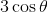to both sides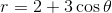### Example Question #122 : Polar Coordinates And Complex Numbers

Which is equivalent to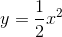?

Possible Answers: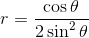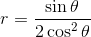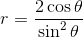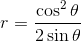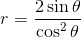Correct answer:Explanation:

To convert, substituteand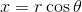: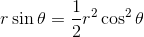divide both sides by r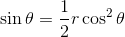multiply both sides by 2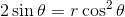divide both sides by cosine squared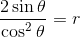### Example Question #123 : Polar Coordinates And Complex Numbers

Convert to polar form: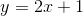Possible Answers: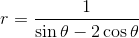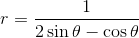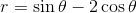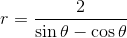Correct answer:Explanation:

Convert by making the substitutionsand: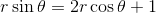subtract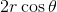from both sides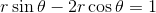factor out r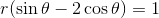divide1 2 3 4 6 Next →

### All Precalculus Resources